# Place value and ordering integers and decimals

### Term 1 starting in week 7 :: Estimated time: 3 weeks

• Recognise the place value of any number in an integer up to one billion
• Understand and write integers up to one billion in words and figures
• Work out intervals on a number line
• Position integers on a number line
• Round integers to the nearest power of ten
• Compare two numbers using =, ?, <, >, ?, ?
• Order a list of integers
• Find the range of a set of numbers
• Find the median of a set of numbers
• Understand place value for decimals
• Position decimals on a number line
• Compare and order any number up to one billion
• Round a number to I significant figure

For higher-attaining pupils:

• Write 10, 100, 1000 etc. as powers of ten
• Write positive integers in the form A x 10n
• Investigate negative powers of ten
• Write decimals in the form A x 10n

This page should remember your ticks from one visit to the next for a period of time. It does this by using Local Storage so the information is saved only on the computer you are working on right now.

## Lesson Starters

Here are some suggestions for whole-class, projectable resources which can be used at the beginnings of each lesson in this block.

### 1st Lesson#### A Number

When written as a word or words what is the smallest positive whole number containing the letter 'a'?

### 2nd Lesson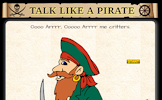#### Numbers in words

Write out in words some numbers writen as digits (optional pirate theme)

### 3rd Lesson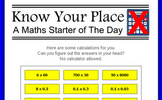Without a calculator perform some calculations requiring a knowledge of place value.

### 4th Lesson#### Writing Cheques

Complete some imaginary cheques, the amount needs to be written in words.

### 5th LessonMake sums from the three digit numbers given.

### 6th Lesson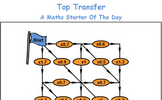#### Top Transfer

Beginning with 100 on your calculator, what is the largest and smallest totals you can end up with after travelling through each of the possible routes.

### 7th Lesson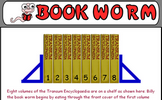#### Book Worm

Work out the distance the book worm needs to eat through to get to the back cover of the last book.

### 8th Lesson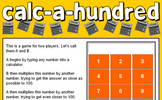#### Calc-A-Hundred

A game for two players requiring a calculator and thinking skills.

### 9th Lesson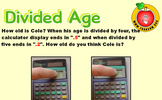#### Divided Age

How old is a person if when her age is divided by certain numbers, the calculator display ending are as shown.

Some of the Starters above are to reinforce concepts learnt, others are to introduce new ideas while others are on unrelated topics designed for retrieval practice or and opportunity to develop problem-solving skills.

White Rose ResourcesEnd of block assessments provide a quick progress check at the end of each block of learning to make sure students have understood the content covered. This Scheme of Learning was produced by White Rose Maths and is used here with permission granted on 30th June 2021.For All: